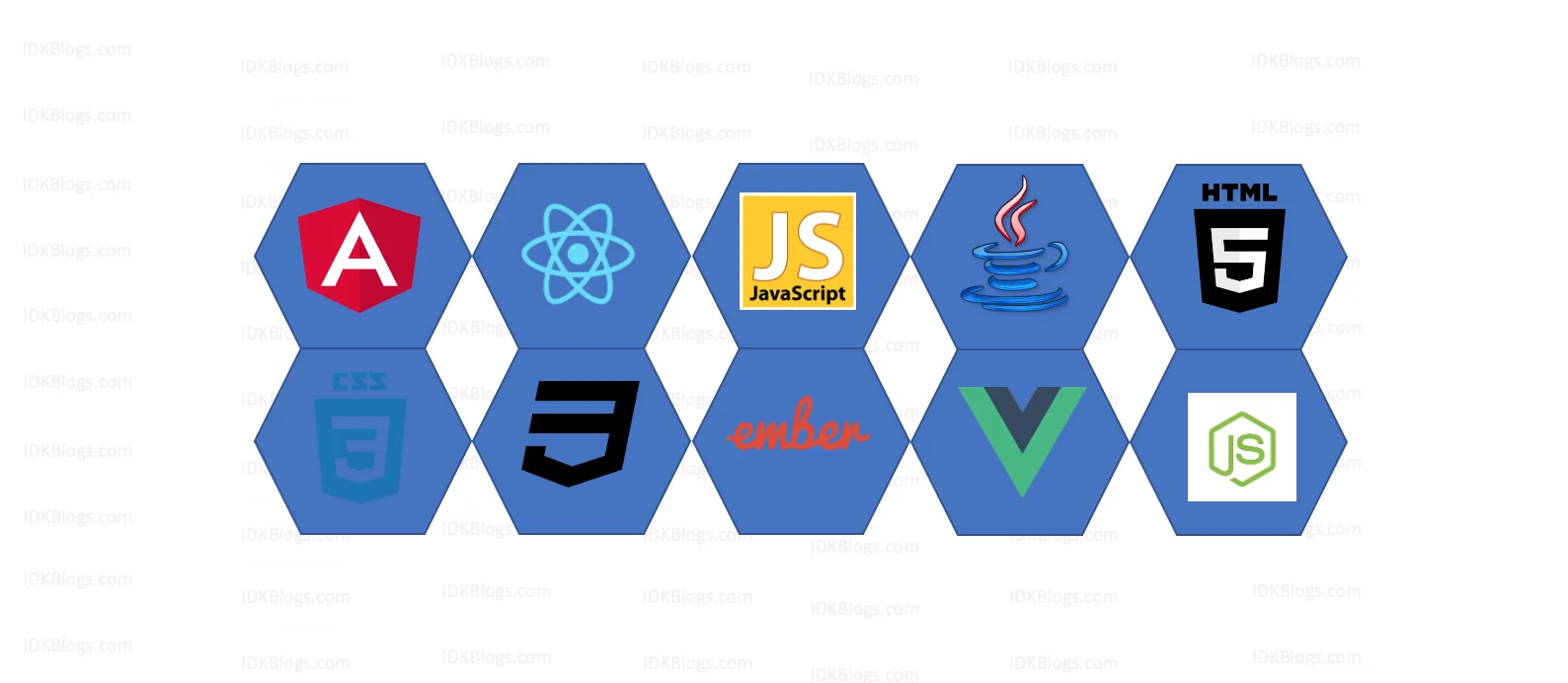# Polyfill for bind() method in JavaScript

### How to make a polyfill for bind method in javaScript

idkblogs.com      July 27, 2021Shubham Verma# Polyfill for bind() method in javaScript

Polyfill is a browser fallback. If your browser don't have the bind() and you have to write your own bind() function, that is what we are going to do here.
So, let's impolement our own bind() function.
Let's have a look of actual bind():

``````                        let name={
fN:'Shubham',
lN:'Verma'
}

let printN=function(){
console.log(`\${this.fN} \${this.lN}`);
}

let printMyN=printN.bind(name);
printMyN();// Shubham Verma
``````
The above code is actual bind that we need to implement our own bind.

Observation:

1. The above code has bind() method which is predefined. we need to create mybind() that will work same as bind() does.

2. printN.bind() is possible then printN.mybind() should be possible, but we need to declare with Function.prototype.mybind().

3. It will return a function, so our mybind() should return a function.

4. let printMyN2=print.mybind(name); and when we call printMyN2(), it should call printN() method with it's this.
So, how we'll get the printN() inside mybind()? we can get this by using this variable.
Inside mybind(),this refers to printN(). this->printN() . So we'll get by let obj=this && obj.call().

5. Now we need to name arguments in mybind().

Let's code:

``````                    Function.prototype.mybind=function(...args){
let obj=this;
return function(){
obj.apply(args);
}
}

let printMyN2 = printN.mybind(name);
printMyN2(); // Shubham Verma
``````

We have done, but the problem is not solved-
If we also want hometown in printN(), then?
let's see.
``````                                let printMyN2 = printN.mybind(name,'Gurgaon');
printMyN2(); // Shubham Verma undefined
``````

So, to get this output, we need to change our code:
``````
let name={
fN:'Shubham',
lN:'Verma'
}

let printN=function(city){
console.log(`\${this.fN} \${this.lN} \${city}`);
}

Function.prototype.mybind=function(...args){
let obj=this;
let params=args.slice(1); // remove 1st element from left and return the rest.
return function(){
obj.apply(args,params);
}
}

let printMyN2 = printN.mybind(name,'Gurgaon');
printMyN2(); // Shubham Verma Gurgaon
``````

Is it done? No, the problem is not yet solved ;(
What if I need to add 'state' of the city.
``````                                let printMyN2 = printN.mybind(name,'Gurgaon');
printMyN2("Haryana"); // Shubham Verma Gurgaon undefined
``````

So, let's fix this:
``````                            let name={
fN:'Shubham',
lN:'Verma'
}

let printN=function(city){
console.log(`\${this.fN} \${this.lN} \${city}`);
}

Function.prototype.mybind=function(...args){
let obj=this;
let params=args.slice(1); // remove 1st element from left and return the rest.
return function(...args2){
obj.apply(args,[...params,...args2]);
}
}

let printMyN2 = printN.mybind(name,'Gurgaon');
printMyN2("Haryana");
``````

Now all set, this will work fine for our mybind().

### Conclusion:

We have learned how we can create our own polyfill for those methods which is not supported by the browsers.

Thank you

To read more about the technologies, Please subscribe us, You'll get the monthly newsletter having all the published article of the last month.

### OTHER POSTS